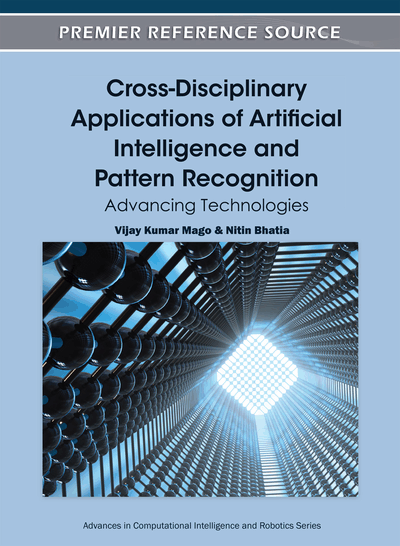# Certain and Uncertain Triangulation in Multiple Camera Vision Systems via LMIs

Graziano Chesi (University of Hong Kong, Hong Kong) and Yeung Sam Hung (University of Hong Kong, Hong Kong)
Copyright: © 2012 |Pages: 12
DOI: 10.4018/978-1-61350-429-1.ch004

## Abstract

Triangulation is a fundamental problem in computer vision that consists of estimating the 3D position of a point of the scene from the estimates of its image projections on some cameras and from the estimates of the projection matrices of these cameras. This chapter addresses multiple view L2 triangulation, i.e. triangulation for vision systems with a generic number of cameras where the sought 3D point is searched by minimizing the L2 norm of the image reprojection error. The authors consider the standard case where estimates of all the image points are available (referring to such a case as certain triangulation), and consider also the case where some of such estimates are not available for example due to occlusions (referring to such a case as uncertain triangulation). In the latter case, it is supposed that the unknown image points belong to known regions such as line segments or ellipses. For these problems, the authors propose a unified methodology that exploits the fundamental matrices among the views and provides a candidate 3D point through the solution of a convex optimization problem based on linear matrix inequalities (LMIs). Moreover, the chapter provides a simple condition that allows one to immediately establish whether the found 3D point is optimal. Various examples with synthetic and real data illustrate the proposed technique, showing in particular that the obtained 3D point is almost always optimal in practice, and that its computational time is indeed small.
Chapter Preview
Top

## Introduction

It is well-known that triangulation is a fundamental problem in computer vision. This problem consists of estimating the 3D position of a point of the scene from its image projections on some cameras and from the projection matrices of these cameras. Triangulation has numerous applications in various areas, for instance it is exploited in the construction of 3D models of real objects from a sequence of images of such objects, procedure known as 3D object reconstruction, see e.g. (Faugeras and Luong (2001), Hung and Tang (2006), Mai et al. (2010)) and references therein. Also, triangulation is exploited in visual servoing for determining the location of a camera mounted on a robot end-effector with respect to a reference object present in the scene: this is realized, firstly, by determining the camera pose between the current and the desired camera locations, and, secondly, by calculating the 3D points of the reference object with respect to the determined projection matrices, see e.g. (Chaumette and Hutchinson (2006), Chaumette and Hutchinson (2007), Chesi and Hashimoto (2010)) and references therein.

In the ideal case where the image projections and projection matrices are exactly known, the triangulation problem can be readily solved, specifically it amounts to solving a system of linear equations (deriving from the projective constraints) in three scalar unknowns, which are the three coordinates of the sought 3D point with respect to an absolute frame used to express the frames of the cameras. The number of equations in this system is equal to twice the number of views, which means that at least two views are required, and that the system is over-determined (i.e., there are more constraints than variables). However, image projections and projection matrices cannot be measured exactly due to image noise, image distortion, etc. This means that the image projections and projection matrices can be only estimated and are hence affected by measurement errors. As a result, the system of linear equations defining the sought 3D point turns out to be infeasible in the general case, i.e. the equations cannot be satisfied for any choice of the three scalar unknowns.

Hence, whenever the data is affected by measurement errors, the problem amounts to finding the 3D point that ``fits’’ the projective constraints better than other 3D points. A possibility consists of looking for the 3D point that minimizes the equation errors, also known as algebraic error. This requires the solution of a linear least-squares minimization, which can be simply obtained either in closed-form or via singular value decomposition (SVD) techniques. However, this criterion may provide unsatisfactory results in some situations since it minimizes an algebraic error rather than a geometric one. In order to cope with this problem, it has been proposed to find the 3D point that minimizes the so-called 2D reprojection error, i.e. the distance between the image projections of the sought 3D point on the camera views and the available measurements.

The 2D reprojection error can be defined in various ways depending on the choice of the distance adopted in the image domain. Typically, this distance is chosen as the standard Euclidean distance since it is known to provide more satisfactory results, and the triangulation is referred to as L2 triangulation. There have been various contributions to the problem of L2 triangulation in the existing literature. In (Hartley and Sturm (1997), Hartley and Zisserman (2000)) the authors show how the exact solution of triangulation with two views can be obtained by computing the roots of a one-variable polynomial of degree six. For triangulation with three-views, the exact solution is obtained in (Stewenius et al. (2005)) by solving a system of polynomial equations through methods from computational commutative algebra, and in (Byrod et al. (2007)) through Groebner basis techniques. Multiple view triangulation is considered for example in (Lu and Hartley (2007)) via branch-and-bound algorithms. See also (Hartley and Seo (2008)) that addresses the problem of verifying global minima and (Bartoli and Lapreste (2008)) that considers the case of points on lines. It is also useful to note some contributions for triangulation with non-L2 norms, such as (Hartley and Schaffalitzky (2004), Ke and Kanade (2006)).

## Complete Chapter List

Search this Book:
Reset